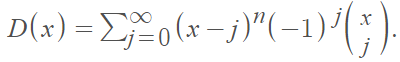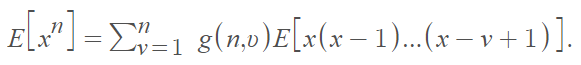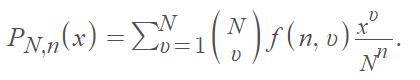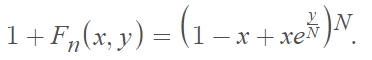# Arfwedson Distribution

Share on

The Arfwedson distribution is a discrete probability distribution for an urn sampling problem for drawings without replacement. Specifically, it tackles the problem “An urn contains N numbered balls. We make n drawings replacing the ball into the urn each time. What is the probability of getting v different balls?” .
The distribution has been known by many names, including:

• The coupon-collecting distribution, because it describes the probability that a person with n randomly selected coupons will have at least one of each of the k equally likely varieties .
• The classical occupancy distribution .
• Stirling2 distribution, because of the presence of the Stirling numbers of the second kind .
• Dixie cup .
• Stevens-Craig [6, 7].
• Arfwedson Distribution Formula.

There are varying formulas in the literature, depending on whether the problem approaches the number of occupied bins or the number of unoccupied bins—which reverses the probability mass function. As an example, Haight lists the Arfwedson distribution asArfwedson  provides the expected value in his 1951 article asWhere the numbers g(n, ν) are Stirling’s second class numbers. One generating function for these is given by

(ex – 1) ν

However, Afrwedson does give another (more complicated) formula as an alternative.

The probability generating function of Arfwedson’s distribution is :Which is equal to the coefficient of yn/n! in the development of this next expression (obtained through integration):References
 G. Arfwedson, A probability distribution connected with Stirling’s second class numbers. Skand. Aktuarietidskr. 34 (1951), 121–132.

 David, F. N., and Barton, D. E. (1962). Combinatorial Chance, London: Griffin. [1.1.3, 10.2, 10.3, 10.4.1, 10.5, 10.6.1]

 O’Neill, B. (2019). The Classical Occupancy Distribution: Computation and Approximation. The American Statistician. n, DOI: 10.1080/00031305.2019.1699445

 Williamson, P. P., Mays, D. P., Abay Asmerom, G., and Yang, Y. (2009), “Revisiting the Classical Occupancy Problem,” The American Statistician, 63, 356–360. [1,2,3]

 Johnson, N. L., and Kotz, S. (1977). Urn Models and Their Application, New York: Wiley. [3.10, 4.2.1, 5.1, 10.4.1, 10.4.2, 11.2.19]

 Stevens, W. L. (1937). Significance of grouping, Annals of Eugenics, London, 8, 57–60. [10.1, 10.4.1]

 Craig, C. C. (1953). On the utilization of marked specimens in estimating populations of flying insects, Biometrika, 40, 170–176. [10.1, 10.4.1]

 Haight, F. (1958). Index to the Distributions of Mathematical Statistics. National Bureau of Standards Report.

CITE THIS AS:
Stephanie Glen. "Arfwedson Distribution" From StatisticsHowTo.com: Elementary Statistics for the rest of us! https://www.statisticshowto.com/arfwedson-distribution/
---------------------------------------------------------------------------Need help with a homework or test question? With Chegg Study, you can get step-by-step solutions to your questions from an expert in the field. Your first 30 minutes with a Chegg tutor is free!